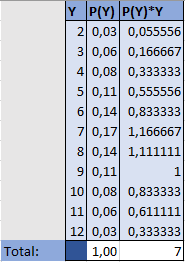The 365 Data Science team is proud to invite you to our own community forum. A very well built system to support your queries, questions and give the chance to show your knowledge and help others in their path of becoming Data Science specialists.The best answers are voted up and moderated by our team

# Probability

0
2

How was the Expected value on the die computed. I will like a break down of it, like what’s the probability of 2, 3, 4 and the rest

365 Team
0

Hey Ilekura,

Thanks for reaching out!

We showcase this in lecture titled The Probability Frequency Distribution around 3:45.

There you’ll see that the probability of each outcome (2, 3, …, 12) equals the number of different ways we can get it over the total number of possible outcomes (36). In some cases, we’ve just simplified the fractions for clarity (e.g. 2/36 is just 1/18 in the table).

Best,
365 Vik

Thanks Vik, but how come the expected value is still 7 of rolling the two die. Using the formula E(value) = P(2)*2+ P(3)*3+…+P(12).12, when I computed it this way I got a decimal. Can you explain this ??

10 months
365 Team
0

Hey Ilekura,

Since I can’t post pictures in a comment, I had to reply to the post again. So, here is a snippet of an Excel file I quickly created where Y showcases all the possible sum of the two dice, P(Y) represents their probabilities of occurring and P(Y)*Y contains their product. At the bottom, you’ll see the sum of all values in the second and third columns respectively, so my guess is you had some computational error in your calculations.Best,
365 Vik

PS: Note that these probabilities are actually written as fractions, but they appear as decimals because the cells are formatted that way.

JanuaryPromo
×
Complete Data Science Education